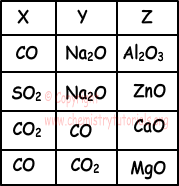## Acids and Bases Exam1 and Problem Solutions

Acids and Bases Exam1 and  Problem Solutions

1. Use following information;

• X and Z react with NaOH and Y does not react with it.
• Y and Z react with HCl but X does not react with it.

Find which one of the followings can be X, Y and Z?Solution:

Acid oxides and amphoteric oxides react with NaOH. Thus, X can not be CO. Since Z reacts with NaOH and HCl it must be amphoteric oxide. It can be Al2O3 and ZnO, since first choice is not true for X, we choose second second one ZnO for Z. Na2O is basic oxides and reacts with HCl. Y becomes Na2O. Thus;

X=SO2

Y=Na2O

Z=ZnO

2. Which ones of the following statements are false for H2SO3?

I. It is produced by reaction of SO2 gas with water.

II. It can form two kinds of salt.

III. 1 mole of H2SO3 react with 1 mole NaOH to neutralization and form salt.

IV. It's conjugate base is HSO3-

Solution:

I. H2SO3 is produced by the following reaction;

SO2 + H2O → H2SO3

I is true

II. It changes one or two of it's H with metal and form salt. II is true.

III. To form neutral salt, it must change all it's H atoms with Na.

H2SO3 + 2NaOH → Na2SO3 + 2H2O

According to this reaction, H2SO3 reacts with 2 mol NaOH to neutralization not 1 mol. III is false.

IV. H2SO3 molecule gives 1 H+ and becomes HSO3- ion. HSO3- is conjugate base of H2SO3. IV is true.

3. pH value of 0,1 M HA solution is 5. Which ones of the following statements are true for this solution?

I. HA is a weak acid.

II. Concentration of OH- ion is 10-9 molar.

Solution:

Since pH of solution is 5, concentration of H+ must be 10-5 molar. Concentration of solution is 0,1 molar, so it is weak acid. Ionization equilibrium of this acid is;

HA(aq)  ↔  H+(aq) + A-(aq)

(0,1-10-5M)   10-5M        10-5M

[H+].[OH-]=10-14 and since [H+]=10-5

[OH-]=10-9

I and II are true.

4. HX and HY have equal volumes and molar concentrations under same temperature. The percentage ionization of HX is larger than HY. Which ones of the followings are larger for HX than HY.

I. Number of moles of dissolved acid.

II. Molar concentration of H+ Ion

III. Electrical conductivity

Solution:

I. Solutions have equal volumes and concentrations, so moles of dissolved acids are also equal. I is false.

II. HX and HY have equal molar concentrations and HX has larger percentage ionization; concentration of H+ ions in HX solutions is larger than HY. II is true.

III. HX has larger percentage ionization, so it had larger amount of ion in same amount of solutions with HY. Electrical conductivity is directly proportional to ion concentration of solution. HX has larger electrical conductivity than HY. III is true.

5. Which ones of the followings are acid-base reaction?

I. NH3(aq) + H3O+(aq) ↔ NH4+(aq) + OH-(aq)

II. Mg(s) + 2H+(aq) ↔ Mg+2(aq) + H2(g)

III. HCO3-(aq) + H2O(l) ↔ CO3-2(aq) + H3O+(aq)

Solution:

I. H3O+gives H+ ion so it is acid and NH3 accepts H+ ion, it is base. It is acid-base reaction.

II. In this reaction there is no H+ ion transfer. Thus it is not acid-base reaction.

III. HCO3-gives H+ ion, so it is acid and H2O accepts H+ ion and it is base. This is acid-base reaction.

Related